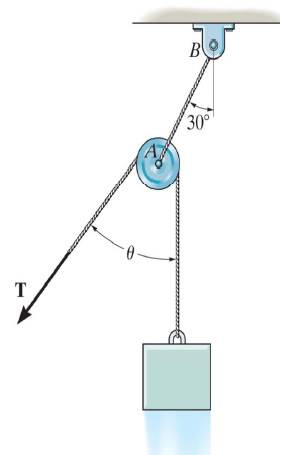# Equilibrium of a particle

#### unknown_2

1. The problem statement, all variables and given/known data
Determine the maximum weight W of the block that can be suspended in the position shown if each cord can support a max tension of 80lb. also what is the angle $$\theta$$ for equilibrium?2. Relevant equations

3. The attempt at a solution
the free body diagram should be:
-force A to B is 60$$^{o}$$ from the positive x axis
-force from the block is in the negative y direction
-tension is in the third quadrant at and angle of $$\theta$$ from the negative y axis

putting the forces in their respected x and y components:
**note: i'm using 30$$^{o}$$ instead of the 60 from the x axis**

F$$_{x}$$ = 80sin30 - Wsin$$\theta$$ = 0
F$$_{y}$$ = 80cos30 - Wcos$$\theta$$ - W = 0

from F$$_{x}$$ : W = $$\frac{80sin30}{sin\theta}$$

sub into F$$_{y}$$:

0=80cos30 - $$\frac{80sin30}{sin\theta}$$cos$$\theta$$ - $$\frac{80sin30}{sin\theta}$$

then i get:
cot30 = $$\frac{cos\theta - 1}{sin\theta}$$
form here i'm not sure how to solve for $$\theta$$. the angle should b 60 deg, but from the solution, it seems to me lyk a random guess....

any help would b appreciated.
cheers
1. The problem statement, all variables and given/known data

2. Relevant equations

3. The attempt at a solution

any1????

#### LowlyPion

Homework Helper
Is θ constrained to be less than 90°?

Because at greater than 90° more weight can be supported it seems, because the Tension of the rope no longer has a negative y component.

### The Physics Forums Way

We Value Quality
• Topics based on mainstream science
• Proper English grammar and spelling
We Value Civility
• Positive and compassionate attitudes
• Patience while debating
We Value Productivity
• Disciplined to remain on-topic
• Recognition of own weaknesses
• Solo and co-op problem solving